# Catalan数推导及应用

Catalan数的定义：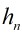表示用下面的方法把凸多边形区域分成三角形区域的方法数：在有n+1条边的凸多边形区域内通过插入在其中不相交的对角线而把它分成三角形区域。定义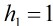。则满足递推关系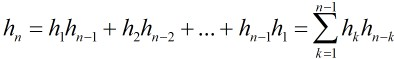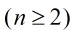再在剩余的n-1个点中选一个点，我们把所选的一条边的两点分别与所选的那一点连接起来，那么多边形被划

分成3部分，一部分有k+1条边，一部分有3条边，另一部分有n-k+1条边，那么这样就划分成了子问题了，所

以按照这个思路可以证明递推式成立。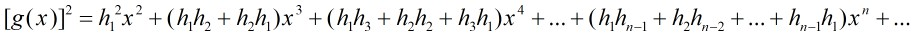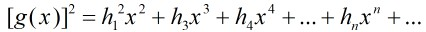，由于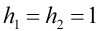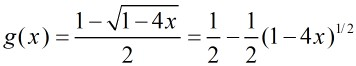，另一个解不符合，舍去。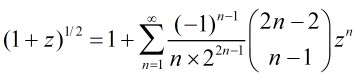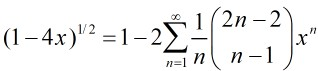1、括号化问题

2、出栈次序问题

a、有2n个人排成一行进入剧场，入场费5元。其中只有n个人有一张5元钞票，另外n人只有10元钞票，剧院无其它钞票，问有多少中方法使得只要有10元的人买票，售票处就有5元的钞票找零？(将持5元者到达视作将5元入栈，持10元者到达视作使栈中某5元出栈)

b、n个1和n个0组成一个2n位的二进制数，要求从左到右扫描，0的累计数不小于1的累计数，求满足条件的的数。

c、12个人排成两排，每排必须是从矮到高排列，而且第二排比对应的第一排的人高，问排列方式有多少种？

010101010101就对应着

3、给定节点组成二叉树的问题

4.n*n棋盘从左下角走到右上角而不穿过主对角线的走法

5.n个+1和n个-1构成的2n项序列，其部分和总满足：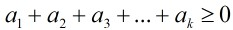的序列的个数。

Catalan数的高精度处理：利用递归式： h(n)=((4*n-2)/(n+1))*h(n-1)

#include <iostream>
#include <stdio.h>
#include <cmath>
using namespace std;

int a;    //大数卡特兰数
int b;         //卡特兰数的长度

void catalan()  //求卡特兰数
{
int i,j,len,carry,temp;
a=b=1;
len=1;
for(i=2;i<=100;i++)
{
for(j=0;j<len;j++)    //乘法
a[i][j]=a[i-1][j]*(4*(i-1)+2);
carry=0;
for(j=0;j<len;j++)    //处理相乘结果
{
temp=a[i][j]+carry;
a[i][j]=temp%10;
carry=temp/10;
}
while(carry)    //进位处理
{
a[i][len++]=carry%10;
carry/=10;
}
carry=0;
for(j=len-1;j>=0;j--) //除法
{
temp=carry*10+a[i][j];
a[i][j]=temp/(i+1);
carry=temp%(i+1);
}
while(!a[i][len-1])     //高位零处理
len--;
b[i]=len;
}
}

int main()
{
int i,n;
catalan();
while(~scanf("%d",&n),n)
{
for(i=b[n]-1;i>=0;i--)
printf("%d",a[n][i]);
printf("\n");
}
return 0;
}


©️2019 CSDN 皮肤主题: 技术黑板 设计师: CSDN官方博客